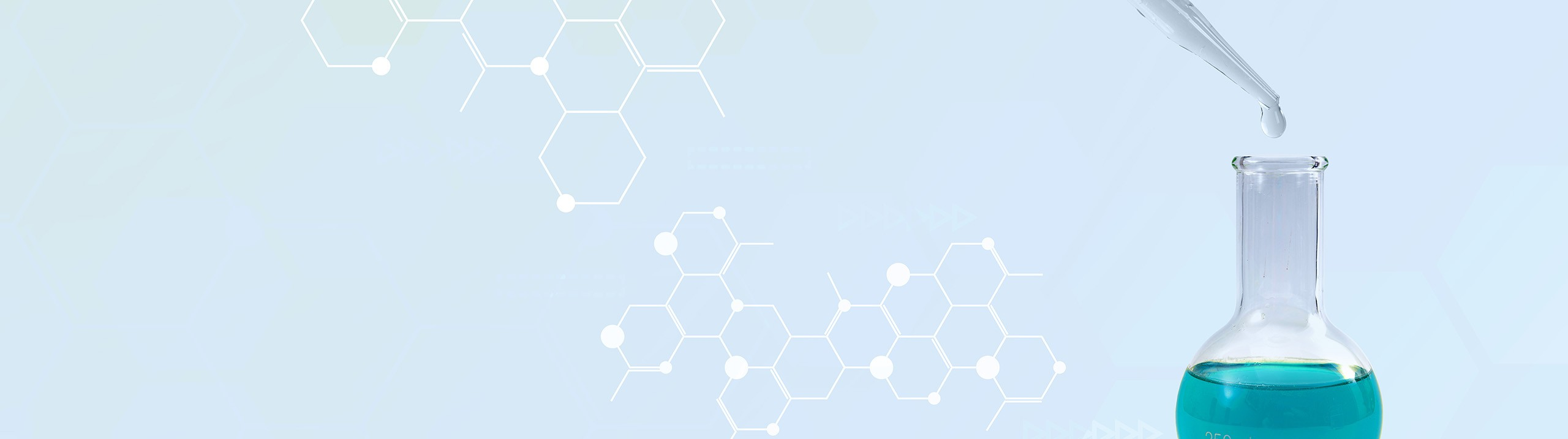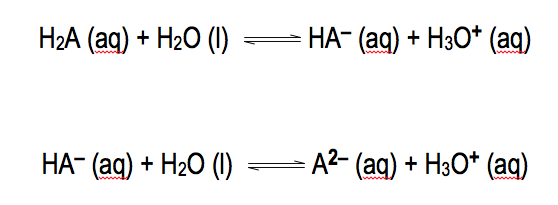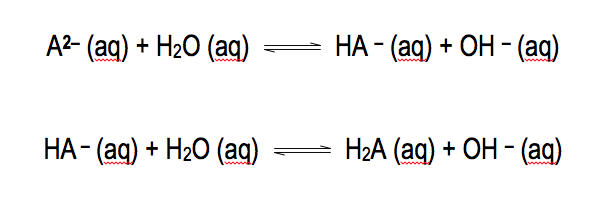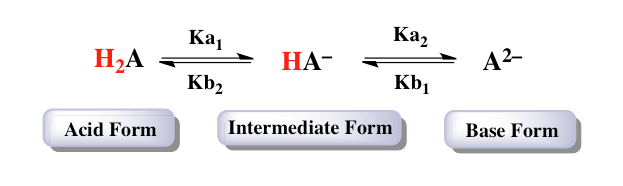## Analytical Chemistry

Learn the toughest concepts covered in your Analytical Chemistry class with step-by-step video tutorials and practice problems.

9. Polyprotic Acid-Base Equilibria

# Diprotic Acids and Bases

Diprotic species involve the movement of two acidic hydrogens.

Diprotic Acids and Bases
1
concept

## Diprotic Acids2m
Play a video:
so die product acids and bases are compounds that could donate or accept two H plus ions. Now for dia protic acid, we're going to see that their basic formula, their generic formula is H two A. And their equations are illustrated by the following two examples. So here when we're dealing with the acid in its fully propagated form, meaning it has both of its acidic hydrogen, it's going to be the acid water is going to be our base. Remember following Bronston Laurie theory, the acid donates an H plus over to the base as a result of this, we create H A minus and H 30. Plus as our products. Since we're talking about removing the first acidic hydrogen from our diet protic acid, that means we're dealing with K. A. one and just like all other K values that equals products overreact ints. So our equilibrium expression would come down to H a minus times H 30. Plus Divided by H two A. Now hear this asset this uh form that we just created which is our conjugate base form because it lost an H. Plus it can continue to lose its second H plus and give us our second equation. So this H a minus continues onward where it still acts as an acid. Another water molecule acts as the base and just like before the acid donates an H. Plus to the base. Now we create a two minus plus H +30. Plus since we're talking about removing the second acidic hydrogen from our dia protic acid. Now it is K. Two that we're dealing with here, we're going to say that this is a two minus times H +30 plus divided by H a minus. Again, we ignore water because in an equilibrium expression we ignore solids and liquids. So these are the two basic equations dealing with the dia protic acid. And before we move on to the dia protic bases, just remember in terms of these equilibrium constants, K one and K two K one is always significantly larger than K. A. To that just means that it's always easiest to remove the first acidic hydrogen from from an acid and it's much more difficult to remove the next one. So remember K one will always be a value much greater than K. A. To click onto the next video and continue our discussion with dia product bases.2
concept

## Diprotic Bases2m
Play a video:
now that we've seen the dia protic acid form of a compound. We can examine its die product base form as well. We're gonna say fur die product bases. They can be represented by a two minus the two minus, representing the fact that it can gain two H plus ions here. The equations can be illustrated by the two examples given below. In this form we have our base form, it hasn't accepted an H. Plus yet. In this first equation, it's the base water is the acid water will donate an H. Plus to it, thereby creating a che minus by donating H plus the water itself becomes O. H. Minus. And because we're talking about accepting the first H plus ion, that means we're dealing with KB. One. Now K. B. one is an equilibrium constant and like all other equilibrium constants, it's equal to products overreact ints. And remember we have to ignore solids and liquids. So here would be H A minus times O. H minus Divided by a 2 -. Now the H A minus that we've created here can continue onward because it's still negative, it can still accept another H. Plus. So we're gonna say here we bring it down. It's still gonna act as the base. It's gonna react with the second water molecule which will act as the acid. Again the water will donate an H plus away to the H A minus, creating H. Two. A. Water again loses an H plus O. Becomes O. H minus. Since we're talking about accepting the second acidic hydrogen. That means we're dealing with K. B. Two. just like the other K. B. It's equal to products. Overreact ints and again, we ignore liquids and solids. So here this would be a church to a times O H minus, divided by H a minus. So at this point we've seen the dye protic acid form, the dye, basic form, die product based form. We've seen K one, K two, K B one and K B two. But how exactly are they connected to one another? By examining the next section, you'll be able to see the connections that we have with these four equilibrium constants as well as the different forms of our Dia product compound. So click on the next video and see how we relate them to one another.3
concept

## Diprotic Dissociation Constants6m
Play a video:
So at this point we've seen the dye protic acid as well as the democratic base in action. And we've seen how we deal with four different equilibrium constants. Now, how exactly do we relate all these things with one another? Well, we're gonna say based on these equations, the relationship between the different forms of diabetic species are we can start out with the fully predominated or acidic form of our diabetic species. So that would be a church to a in its asset form. It only has one choice. It can choose to donate an H plus away by giving away that first H plus. That gives us a church a minus. And since we're talking about losing the first acidic H plus, that means we're dealing with K. A one now, H A minus. Which represents our intermediate form, can also decide to donate an H plus away giving us a two minus. Since we're talking about removing the second acidic hydrogen, that means we're dealing with K. A. Two. But remember these die product species, we can also look look at them in terms of a base. So here for this basic species here, we can say that it accepts its first H plus to become a church a minus. By accepting that first acidic hydrogen. That means we're dealing with KB one then the intermediate H a minus can decide to accept another H plus to become a church to a By accepting that second acidic hydrogen. That means we're dealing with K. B two. Now notice we have here, K K one and K B two, overlapping one another. And what do they do? They relate the acid form to the intermediate form, then we also have a on K. Two and K. B one overlapping one another. And they relate the basic form with the intermediate form. Because of this, we can establish relationships between K and K. B. So, because of the overlapping, we can say here that K one times K. B two gives us K. W. And we can also say because of the overlapping K two times KB one equals Kw. Remember K W represents the dissociation constant for water, which is 1.0 times 10 to the negative 14 when the temperature is 25 degrees Celsius. Remember all equilibrium constants are temperature dependent if we change the temperature, K W would change if they did that. They would tell you what the new K W would be. Now, because of this, we can establish some guidelines when dealing with one of these forms of our diet product species. So, if we take a look, we're gonna say here, if we're dealing with htwe, this is the fully propagated acidic form treated as you would a mono product acid. So we'd say here that we would use K one when dealing with it in order to find ph we'll skip the intermediate form for now and come back to it. H two minus has no H plus on it. So it only has one choice to accept an H plus and act like a base because we're accepting our first H plus. That means that it's going to act as a basic use KB one. Now the intermediate form is tricky because the intermediate form here can do one of two things. It could either donate another H plus and become The basic form and in that case would use K. A. two or it could decide to gain another H plus to become the acid form and therefore use KB two. So for this one it could use K two or K. B two. So it really depends on the species itself. Now realize this intermediate form has a hydrogen so it can act as an acid and a base because it's a negative charge there if you can act as an acid or base. Remember you're called an Amfa Terek species. Now, ample Terex species. We have acidic ones and basic ones. The acidic ones would donate H Plus and therefore use K two. So here we have hydrogen sulfate, we'd have hydrogen sulfite or by sulfate and by cell fight and then we have here di hydrogen phosphate. So these are your most common amfa Terek acidic compounds that would use your K two. And technically here this one fits into poly product because it has more than one. Um we'll talk about that later on in our polity product assets video then we have our basic ones. So these basic ones here would use KB two. So who fits in here? We have hydrogen carbonate, also called bicarbonate. We have hydrogen sulfide and then we have hydrogen phosphate. Again, this falls into the arena arena of polyp product species. So for right now, don't worry about these two here. Just realized later on when we talk about um philatelic species that they themselves represent acidic Amfa Terek and basic and eric species. So again, remember when it comes to the intermediate form, it could be K two or K B two, depending on if it wants to act as an acid or as a base respectively. And in terms of this topic, when we're talking about di product species, remember the first two that I listed for acidic and the first toy listed for basic. Those are the um categories they fall into and determine if they use K two or K B. To hopefully guys were able to follow along in terms of this now that we've gotten out of the way all the theory and ideas behind dive product species will continue onward with calculations in terms of finding ph and concentrations of these different types of compoundsDiprotic Acids and Bases Calculations
4
example

## Diprotic Acids and Bases Calculations 113m
Play a video:
5
example

## Diprotic Acids and Bases Calculations 15m
Play a video:
here it states determine the ph of a 0.80 Mueller sodium sulfide solution. Now, here we're told that hydro sulfuric acid which is H. Two S contains K. One equal to 1.0 times 10 to the negative seven and K two equal to 9.1 times 10 to the negative eight. Now realize here that we're dealing with sodium sulfide, that's the compound we have to work with. The only reason we're giving information on hydro sulfuric acid is so that we can use its K. One and K. Two values realize here that sodium sulfide has had all of its hydrogen is removed. So sodium sulfide represents the basic form of our diaper tick acid here, it would represent to sodium and then the sulfide ion, the sulfide ion represents our basic form of our die protic acid. We can ignore the sodium because it's just a spectator ions. So we're gonna bring down the sulfide ion that we have. It's gonna react with water here, it's the base. So water is going to be the acid, the acid will donate an H plus over to the base to give us a church S minus plus O. H minus. We have initial change equilibrium. Remember in a nice chart, we ignore solids and liquids. So we're gonna ignore the liquid water. Our initial concentration is .080 moller. These initially are zero. We're gonna lose our reactant. So minus X. To help build up our products plus X plus X. Bring down everything 0.80 minus X plus X plus X. Now realize here, we're talking about accepting the first acidic hydrogen by the sulfide ion because we're accepting the first acidic hydrogen. That means we're dealing with KB one. But here, what we have we have K one and K two. What's the connection that KB one has? Well remember earlier we said that K B one and K two are connected together. And when you multiply them together, that gives us K. W. Alright, so We just have to isolate KB one. So KB one equals KW Divided by K two. So 1.0 times 10 to the -14, divided by 9.1 times 10 to the -8. When we do that, that's gonna give me my KB one value as 1.1 times 10 to the negative seven. So that's our KB one that will be utilizing. So we're gonna come over here. So KB one just like other equilibrium constants equals products. Overreact ints. Now we're gonna input the values we know for each. So 1.1 times 10 to the negative seven equals X squared divided by 70.80 minus x. We have to check to see can I ignore that minus X or not? By doing a 5% approximation method. So we're gonna take the initial concentration that we're dealing with divided by the equilibrium constant that we're utilizing, which is K B one. And look to see if that ratio is greater than 500. So here's our initial concentration Divided by the KB one that we're using. So that ratio is definitely greater than 500. So that means that this minus X is not significant enough to do any real change. So we ignore it. Alright. So now we just have to solve for X right now. So we're gonna multiply both sides by zero, So here X squared equals 8.8 times 10 to the negative. Nine. Take the square root of both sides here. So that gives me x equals 9.4 times 10 to the -5. Now, anytime you find X, it's gonna give you either H 30 plus or minus this X. That we isolated helps to give us a way to minus because we know the concentration of a hydroxide ion. We can determine P. O. H. Because P. O. H is just a negative log of hydroxide ion. So that equals 4.03. And because we know that P. H. equals 14 -4.03. So that's going to be 9.97 as our p. So the key to solving these types of dye product species questions is to determine are you dealing with the acid form, Are you dealing with the acidic form? Are you dealing with the intermediate form in the two examples on this page, we've seen what to do when asked to determine the ph of the acid form and the basic form. Later on, we'll see what do we do when dealing with the intermediate form of a dia product species.
6
example

## Diprotic Acids and Bases Calculations 24m
Play a video: